# Auto-Spectral Density (ASD)

June 2, 2021

The auto-spectral density function is the discrete-time Fourier transform of the auto-correlation function Rxx(m). It is also known as the power spectral density (PSD).

The auto-spectral density function is defined as:

(1)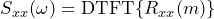(2)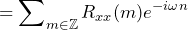The PSD expresses how correlated a signal is in relation to itself. A signal with a flat PSD indicates that it is very uncorrelated whereas a signal with a main lobe has some degree of correlation.

Generally, the wider the main lobe, the more uncorrelated the signal is. The narrower the main lobe, the more correlated it is.

#### Examples

1. If a PSD is flat, then the samples in the sequence are uncorrelated. In the case of white noise, the PSD equals 1 for all frequencies. White noise in signal analysis, which has equal power density at each frequency, corresponds to white light in physics, which has equal power density at each frequency.
2. A PSD with a single spike at 0Hz is likely caused by the presence of a large DC offset in the signal.
3. A PSD with a single spike at some non-zero frequency (F) in Hertz likely indicates that a signal is correlated with itself at regular intervals of 1/F seconds. This correlation may be caused by a resonant frequency at F.
4. The wider the main lobe, the more uncorrelated the adjacent samples. A constant PSD with an infinite main lobe width is completely uncorrelated and indicates that the signal is white noise.

### Estimating the ASD

In a real-world test, the true ASD cannot be known nor calculated. However, if an ASD function is not changing with time—as in, the data are stationary—then the true PSD can be estimated.

From the definition of ASD, we can determine that Sxx(ω) depends on two operators: DTFT operator F and expectation operator E.

The auto-spectral density function is defined as:

 (3)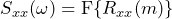by definition of Sxx(ω) (4)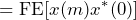by definition of Rxx(m)

In general, linear operators are not commutative. That is, FE = EF is not generally true. In the case of wide-sense stationary random sequences, however, we can estimate Sxx(ω) by allowing F and E to commute:

 (5)by definition of Sxx (6)by definition of Rxx(m) (7)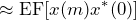if wide-sense stationary

(8)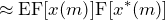(9)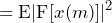The Fourier transform F of the random sequence x(m) is random. However, when we take the expectation E of the sequence, it becomes deterministic.

The expectation E can be estimated by computing the arithmetic mean. Therefore, computing the average of the sequence’s absolute Fourier transforms squared is a method of estimating the ASD.

In practice, however, it has been found that first smoothing the data using windowing is necessary for better estimation.

### Welch’s Method

There are several standard mathematical methods of estimating ASD that date back to the 1890s. All these methods depend on collecting samples and then calculating an estimate based on those samples.

The above discussion describes a method of ASD estimation called Welch’s method. It is based on a 1967 paper by Peter Welch and is perhaps the most common method for PSD estimation in the industry.

In Welch’s method, the data sequence is divided into several analysis frames of equal length that are windowed with a window function. The windowed frames can overlap.

A fast Fourier transform (FFT) is performed on each frame, and each element of the FFT operations is squared (consistent with the idea of power). Finally, the squared FFT segments are averaged to yield an estimated PSD.

### ASD and Spectral Power

Parseval’s theorem tells us that power can be computed in time by calculating the sum of the squares of the time samples or in frequency by calculating the sum of the squared frequency samples.

The above discussion demonstrates that ASD can be estimated by calculating the average of the sum of the squares of the frequency samples. By Parseval’s theorem, this is, in essence, equivalent to spectral power. It explains why the auto-spectral density is also called the PSD.

In real-world analysis, environments with physical shaking use a resonant peak to represent the energy/power at that frequency.